## Pandas, Numpy, JSON & SSL (Crossover of Space Stone & Reality Stone in Python Verse)

In our last installment, we’ve shown pandas & numpy based on a specific situation. If that is our Space Stone installment of Python Verse, then this would be one approach of creating much interesting crossover of Space Stone & Reality Stone of Python verse. Yes. You are right. We’ll be discussing one requirement, where we need many of these in a single task.

Let’s dive into it!

Let’s assume that we have a source csv file which has the following data –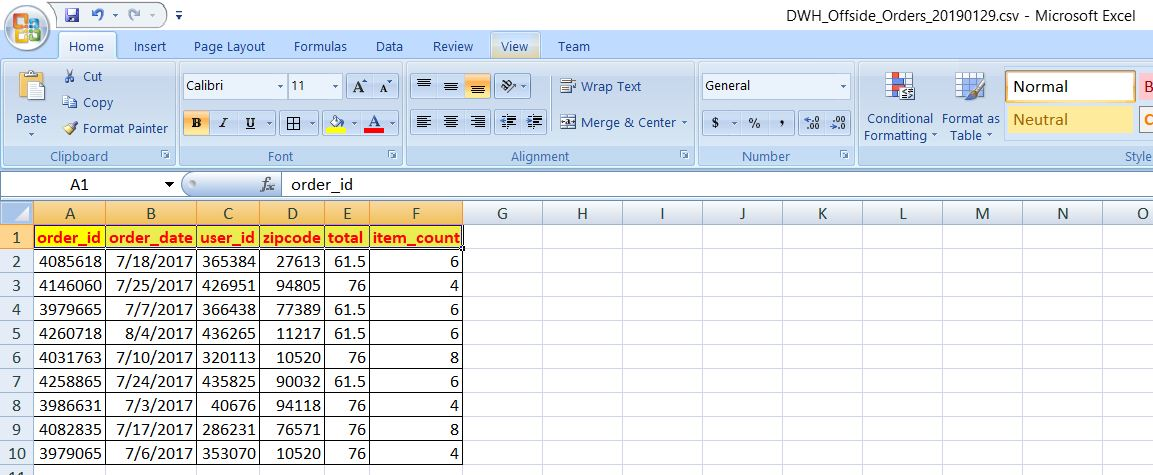Now, the requirement is – we need to use one third party web service to send JSON payload preparing with this data & send them to the 3rd party API to get the City, State & based on that we need to find the total number of item sold against each State & City.Let’s look into our third-party API site  –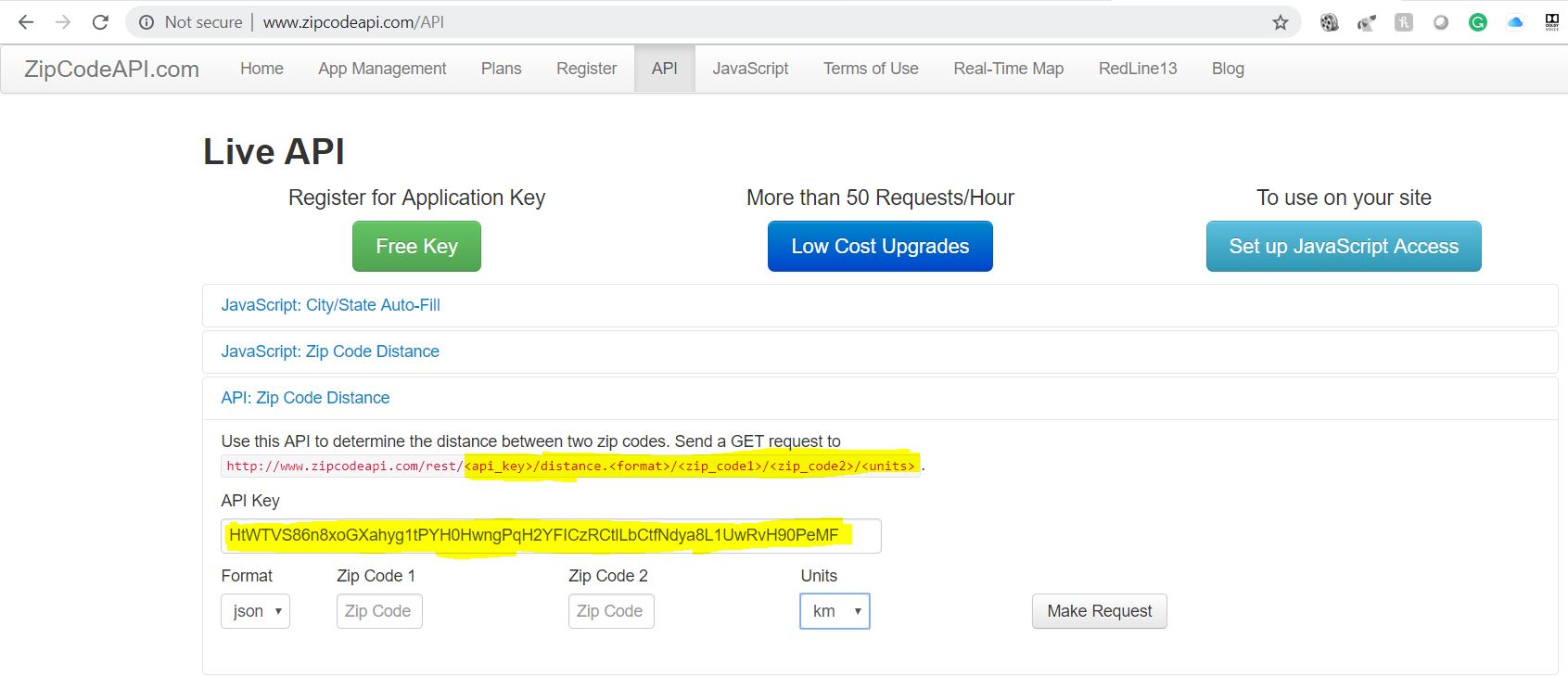As per the agreement with this website, any developer can test 10 calls per day free. After that, it will send your response with encrypted values, e.g. Classified. But, we don’t need more than 10 calls to test it.

Here, we’ll be dealing with the 4 python scripts. Among them, one scenario I’ve already described in my previous post. So, I’ll be just mentioning the file & post the script.

Please find the directory structure in both the OS –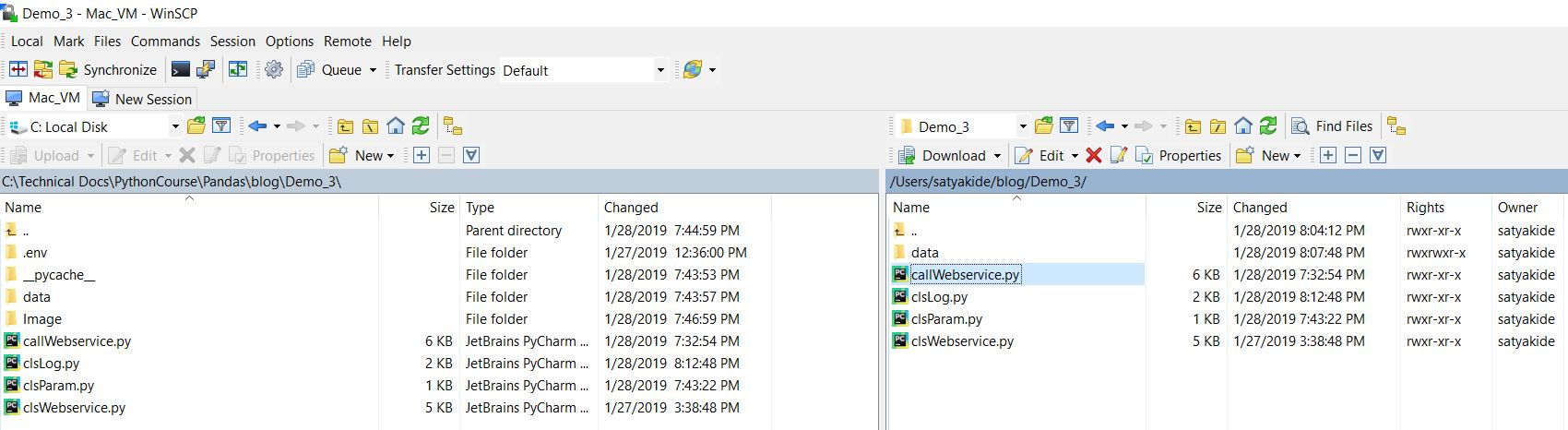1. clsLpy (This script will create the split csv files or final merge file after the corresponding process. However, this can be used as usual verbose debug logging as well. Hence, the name comes into the picture.)

 ``` 1 2 3 4 5 6 7 8 9 10 11 12 13 14 15 16 17 18 19 20 21 22 23 24 25 26 27 28 29 30 31 32 33 34 35 36 37 38 39 40 41``` ```########################################### #### Written By: SATYAKI DE ######## #### Written On: 20-Jan-2019 ######## ########################################### import pandas as p import os import platform as pl from clsParam import clsParam as cf class clsL(object): def __init__(self): self.path = cf.config['PATH'] def logr(self, Filename, Ind, df, subdir=None): try: x = p.DataFrame() x = df sd = subdir os_det = pl.system() if sd == None: if os_det == "Windows": fullFileName = self.path + '\\' + Filename else: fullFileName = self.path + '/' + Filename else: if os_det == "Windows": fullFileName = self.path + '\\' + sd + "\\" + Filename else: fullFileName = self.path + '/' + sd + "/" + Filename if Ind == 'Y': x.to_csv(fullFileName, index=False) return 0 except Exception as e: y = str(e) print(y) return 3 ```

2. clsParam.py (This script contains the parameter entries in the form of dictionary & later this can be used in all the relevant python scripts as configuration parameters.)

 ``` 1 2 3 4 5 6 7 8 9 10 11 12 13 14 15``` ```########################################### #### Written By: SATYAKI DE ######## #### Written On: 20-Jan-2019 ######## ########################################### import os class clsParam(object): config = { 'MAX_RETRY' : 5, 'API_KEY' : 'HtWTVS86n8xoGXahyg1tPYH0HwngPqH2YFICzRCtlLbCtfNdya8L1UwRvH90PeMF', 'PATH' : os.path.dirname(os.path.realpath(__file__)), 'SUBDIR' : 'data' } ```

As you can see from this script that we’ve declared all the necessary parameters here as dictionary object & later we’ll be referring these parameters in the corresponding python scripts.

`'API_KEY' : 'HtWTVS86n8xoGXahyg1tPYH0HwngPqH2YFICzRCtlLbCtfNdya8L1UwRvH90PeMF'`

One crucial line, we’ll look into. API_KEY will be used while sending the JSON payload to the third-party web service. We’ll get this API_KEY from the highlighted (In Yellow) picture posted above.

3. clsWeb.py (This is the main script, which will first convert the pandas’ data frames into JSON & and send the API request as per the third party site.  It will capture the response & convert that by normalizing the data & poured it back to the data frame for further process.)

 ``` 1 2 3 4 5 6 7 8 9 10 11 12 13 14 15 16 17 18 19 20 21 22 23 24 25 26 27 28 29 30 31 32 33 34 35 36 37 38 39 40 41 42 43 44 45 46 47 48 49 50 51 52 53 54 55 56 57 58 59 60 61 62 63 64 65 66 67 68 69 70 71 72 73 74 75 76 77 78 79 80 81 82 83 84 85 86 87 88 89 90 91 92 93 94 95 96 97 98 99 100 101 102 103 104 105 106 107 108 109 110 111 112 113 114 115 116 117 118 119 120 121 122 123 124 125 126 127 128 129 130 131 132 133 134 135 136 137 138 139 140 141 142 143 144 145 146 147 148 149 150``` ```########################################### #### Written By: SATYAKI DE ######## #### Written On: 20-Jan-2019 ######## ########################################### import json import requests import datetime import time import ssl from urllib.request import urlopen import pandas as p import numpy as np import os import gc from clsParam import clsParam as cp class clsWeb(object): def __init__(self, payload, format, unit): self.payload = payload self.path = cp.config['PATH'] # To disable logging info self.max_retries = cp.config['MAX_RETRY'] self.api_key = cp.config['API_KEY'] self.unit = unit self.format =format def get_response(self): # Assigning Logging Info max_retries = self.max_retries api_key = self.api_key unit = self.unit format = self.format df_conv = p.DataFrame() cnt = 0 try: # Bypassing SSL Authentication try: _create_unverified_https_context = ssl._create_unverified_context except AttributeError: # Legacy python that doesn't verify HTTPS certificates by default pass else: # Handle target environment that doesn't support HTTPS verification ssl._create_default_https_context = _create_unverified_https_context # Capturing the payload data_df = self.payload temp_df = data_df[['zipcode']] list_of_rec = temp_df['zipcode'].values.tolist() print(list_of_rec) for i in list_of_rec: zip = i # Providing the url url_part = 'http://www.zipcodeapi.com/rest/' url = url_part + api_key + '/' + 'info.' + format + '/' + str(zip) + '/' + unit headers = {"Content-type": "application/json"} param = headers var1 = datetime.datetime.now().strftime("%H:%M:%S") print('Json Fetch Start Time:', var1) retries = 1 success = False while not success: # Getting response from web service response = requests.get(url, params=param, verify=False) # print("Complete Error:: ", str(response.status_code)) # print("Error First::", str(response.status_code)[:1]) if str(response.status_code)[:1] == '2': # response = s.post(url, params=param, json=json_data, verify=False) success=True else: wait = retries * 2 print("Retry fails! Waiting " + str(wait) + " seconds and retrying.") time.sleep(wait) retries += 1 # Checking Maximum Retries if retries == max_retries: success=True raise ValueError # print(response.text) var2 = datetime.datetime.now().strftime("%H:%M:%S") print('Json Fetch End Time:', var2) print("-" * 90) # Capturing the response json from Web Service df_response_json = response.text string_to_json = json.loads(df_response_json) # Converting the response json to Dataframe # df_Int_Rec = p.read_json(string_to_json, orient='records') df_Int_Rec = p.io.json.json_normalize(string_to_json) df_Int_Rec.columns = df_Int_Rec.columns.map(lambda x: x.split(".")[-1]) if cnt == 0: df_conv = df_Int_Rec else: d_frames = [df_conv, df_Int_Rec] df_conv = p.concat(d_frames) cnt += 1 # Deleting temporary dataframes & Releasing memories del [[df_Int_Rec]] gc.collect() # Resetting the Index Value df_conv.reset_index(drop=True, inplace=True) # Merging two data side ways maintaining the orders df_add = p.concat([data_df, df_conv], axis=1) del [[df_conv]] gc.collect() # Dropping unwanted column df_add.drop(['acceptable_city_names'], axis=1, inplace=True) return df_add except ValueError as v: print(response.text) x = str(v) print(x) # Return Empty Dataframe df = p.DataFrame() return df except Exception as e: print(response.text) x = str(e) print(x) # Return Empty Dataframe df = p.DataFrame() return df ```

Let’s look at the key lines to discuss –

```def __init__(self, payload, format, unit):

self.path = cp.config['PATH']

# To disable logging info
self.max_retries = cp.config['MAX_RETRY']
self.api_key = cp.config['API_KEY']
self.unit = unit
self.format = format```

The first block will be instantiated as soon as you are invoking the class. Note that, we’ve used our parameter class python script here as cp & then we’re referring the corresponding elements as & when requires. Other parameters will be captured from the invoking script, which we’ll be discussed later in this post.

```# Bypassing SSL Authentication
try:
_create_unverified_https_context = ssl._create_unverified_context
except AttributeError:
# Legacy python that doesn't verify HTTPS certificates by default
pass
else:
# Handle target environment that doesn't support HTTPS verification
ssl._create_default_https_context = _create_unverified_https_context```

Sometimes, Your Firewall or Proxy might block your web service request due to a specific certificate error. This snippet will bypass that authentication. However, it is always advised to use proper SSL certification in the Production environment.

```# Capturing the payload
temp_df = data_df[['zipcode']]

list_of_rec = temp_df['zipcode'].values.tolist()```

In this snippet, we’re capturing the zip code from our source data frame & converting them into a list & this would be our candidate to pass the data as part of our JSON payload.

```for i in list_of_rec:
zip = i

# Providing the url
url_part = 'http://www.zipcodeapi.com/rest/'
url = url_part + api_key + '/' + 'info.' + format + '/' + str(zip) + '/' + unit

Once, we’ve extracted our zip codes, we’re passing it one-by-one & forming our JSON with header & data.

```retries = 1
success = False

while not success:
# Getting response from web service
response = requests.get(url, params=param, verify=False)
# print("Complete Error:: ", str(response.status_code))
# print("Error First::", str(response.status_code)[:1])

if str(response.status_code)[:1] == '2':
# response = s.post(url, params=param, json=json_data, verify=False)
success=True
else:
wait = retries * 2
print("Retry fails! Waiting " + str(wait) + " seconds and retrying.")
time.sleep(wait)
retries += 1

# Checking Maximum Retries
if retries == max_retries:
success=True
raise ValueError```

In this section, we’re posting our JSON application & waiting for the response from the third-party API. If we receive the success response (200), we will proceed with the next zip code. However, if we didn’t receive the success response, we’ll retry the post option again until or unless it reaches the maximum limits. In case, if the application still waiting for a valid answer even after the maximum limit, it will exit from the loop & raise an error to the main application.

```# Capturing the response json from Web Service
df_response_json = response.text

# Converting the response json to Dataframe
df_Int_Rec = p.io.json.json_normalize(string_to_json)
df_Int_Rec.columns = df_Int_Rec.columns.map(lambda x: x.split(".")[-1])```

This snippet will extract the desired response from the API & convert that back to the Pandas data frame. Last two lines, it is normalizing the data that it has received from the API for further process. This is critical steps as these steps will lead to extract City & State from our API response.

```# Merging two data side ways maintaining the orders

Once, we’ll have structured data – we can merge it back to our source data frame for our next step.

4. callWebservice.py (This script will call the API script & also process the data to create an aggregate report for our task.)

 ``` 1 2 3 4 5 6 7 8 9 10 11 12 13 14 15 16 17 18 19 20 21 22 23 24 25 26 27 28 29 30 31 32 33 34 35 36 37 38 39 40 41 42 43 44 45 46 47 48 49 50 51 52 53 54 55 56 57 58 59 60 61 62 63 64 65 66 67 68 69 70 71 72 73 74 75 76 77 78 79 80 81 82 83 84 85 86 87 88 89 90 91 92 93 94 95 96 97 98 99 100 101 102 103 104 105 106 107 108 109 110 111 112 113 114 115 116 117 118 119 120 121 122 123 124 125 126 127 128 129 130 131 132 133 134 135 136 137 138 139 140 141 142 143 144 145 146 147 148 149 150 151 152 153 154``` ```##################################################### ### Objective: Purpose of this Library is to call ### ### the Web Service method to capture the city, ### ### & the state as a json response & update them ### ### in the dataframe & finally produce the summary### ### of Total Sales & Item Counts based on the City### ### & the State. ### ### ### ### Arguments are as follows: ### ### Mentioned the Exception Part. First time dry ### ### run the program without providing any args. ### ### It will show all the mandatory params. ### ### ### ##################################################### ##################################################### #### Written By: SATYAKI DE ### #### Written On: 20-Jan-2019 ### ##################################################### import clsWeb as cw import sys import pandas as p import os import platform as pl import clsLog as log import datetime import numpy as np from clsParam import clsParam as cp # Disbling Warnings def warn(*args, **kwargs): pass import warnings warnings.warn = warn def main(): print("Calling the custom Package..") try: if len(sys.argv) == 4: inputFile = str(sys.argv) format = str(sys.argv) unit = str(sys.argv) else: raise Exception # Checking whether the format contains # allowable choices or not if (format == 'JSON'): format = 'json' elif (format == 'CSV'): format = 'csv' elif (format == 'XML'): format = 'xml' else: raise Exception # Checking whether the format contains # allowable choices or not if (unit == 'DEGREE'): unit = 'degree' elif (unit == 'RADIANS'): unit = 'radians' else: raise Exception print("*" * 170) print("Reading from " + str(inputFile)) print("*" * 170) # Initiating Logging Instances clog = log.clsLog() path = cp.config['PATH'] subdir = cp.config['SUBDIR'] os_det = pl.system() if os_det == "Windows": src_path = path + '\\' + 'data\\' else: src_path = path + '/' + 'data/' # Reading source data csv file df_Payload = p.read_csv(src_path+inputFile, index_col=False, skipinitialspace=True) x = cw.clsWeb(df_Payload, format, unit) retDf = x.get_response() # Total Number of rows fetched count_row = retDf.shape if count_row == 0: print("Data Processing Issue!") else: print("Writing to file -> (" + str(inputFile) + "_modified.csv) Status: Success") FileName, FileExtn = inputFile.split(".") # Writing to the file clog.logr(FileName + '_modified.' + FileExtn, 'Y', retDf, subdir) print("*" * 170) # Performing group by operation to get the desired result # State & City-wise total Sales & Item Sales df_src = p.DataFrame() df_src = retDf[['city', 'state', 'total', 'item_count']] # Converting values to Integer df_src['city_1'] = retDf['city'].astype(str) df_src['state_1'] = retDf['state'].astype(str) df_src['total_1'] = retDf['total'].astype(int) df_src['item_count_1'] = retDf['item_count'].astype(int) # Dropping the old Dtype Columns df_src.drop(['city'], axis=1, inplace=True) df_src.drop(['state'], axis=1, inplace=True) df_src.drop(['total'], axis=1, inplace=True) df_src.drop(['item_count'], axis=1, inplace=True) # Renaming the new columns to as per Old Column Name df_src.rename(columns={'city_1': 'city'}, inplace=True) df_src.rename(columns={'state_1': 'state'}, inplace=True) df_src.rename(columns={'total_1': 'total'}, inplace=True) df_src.rename(columns={'item_count_1': 'item_count'}, inplace=True) # Performing Group By Operation grouped = df_src.groupby(['state', 'city']) res_1 = grouped.aggregate(np.sum) print("DF:") print(res_1) FileName1 = 'StateCityWiseReport' # Handling Multiple source files var = datetime.datetime.now().strftime(".%H.%M.%S") print('Target File Extension will contain the following:: ', var) # Writing to the file clog.logr(FileName1 + var + '.' + FileExtn, 'Y', df_src, subdir) print("*" * 170) print("Operation done for " + str(inputFile) + "!") print("*" * 170) except Exception as e: x = str(e) print(x) print("*" * 170) print('Current order would be - <' + str(sys.argv) + '> ') print('Make sure last two params should be in CAPS only!') print("*" * 170) if __name__ == "__main__": main() ```

Let’s look at some vital code snippet in this main script –

```# Reading source data csv file

retDf = x.get_response()```

In this snippet, we’re getting our data from our source csv & then calling our leading Web API service to get the State & City information.

```# Converting values to Integer
df_src['city_1'] = retDf['city'].astype(str)
df_src['state_1'] = retDf['state'].astype(str)
df_src['total_1'] = retDf['total'].astype(int)
df_src['item_count_1'] = retDf['item_count'].astype(int)```

Converting individual data type to appropriate data types. In Pandas, it is always advisable to change the data type of frames to avoid unforeseen scenarios.

```# Dropping the old Dtype Columns
df_src.drop(['city'], axis=1, inplace=True)
df_src.drop(['state'], axis=1, inplace=True)
df_src.drop(['total'], axis=1, inplace=True)
df_src.drop(['item_count'], axis=1, inplace=True)

# Renaming the new columns to as per Old Column Name
df_src.rename(columns={'city_1': 'city'}, inplace=True)
df_src.rename(columns={'state_1': 'state'}, inplace=True)
df_src.rename(columns={'total_1': 'total'}, inplace=True)
df_src.rename(columns={'item_count_1': 'item_count'}, inplace=True)```

Now, dropping the old columns & renaming the new columns to get the same column with correct data types. I personally like this way as it is an immaculate way to do this task. You can also debug it easily.

```# Performing Group By Operation
grouped = df_src.groupby(['state', 'city'])
res_1 = grouped.aggregate(np.sum)```

And, finally, using Pandas group-by method we’re aggregating the groups & then using numpy to generate the same against each group.

Please check the first consolidated output –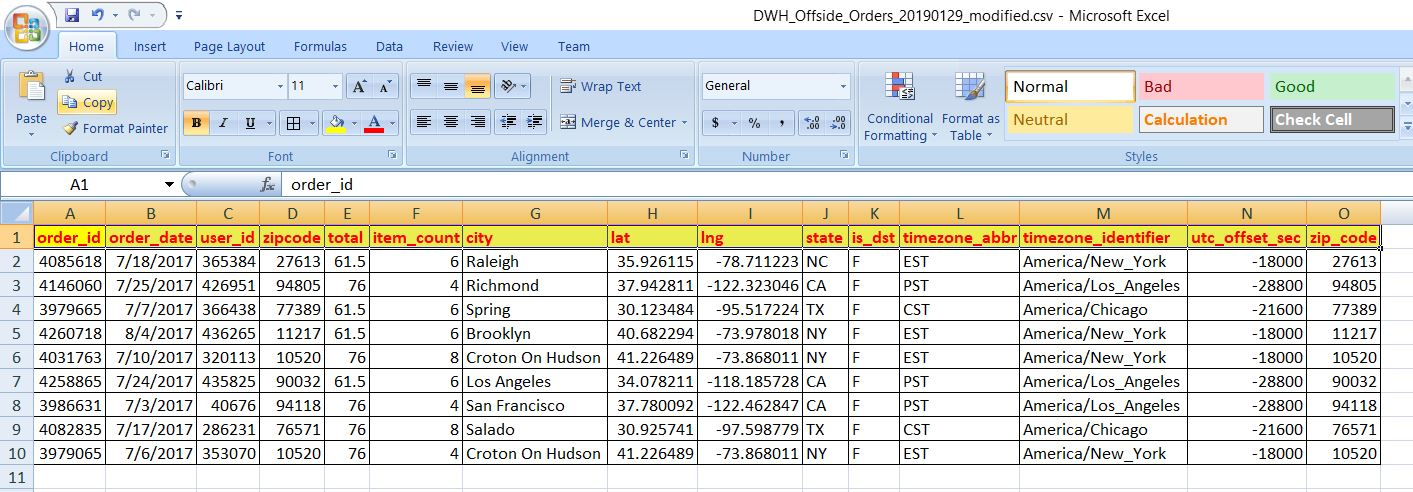From this screenshot, you can see how we have the desired intermediate data of City & State to proceed for the next level.

Let’s see how it runs –

Windows (64 bit):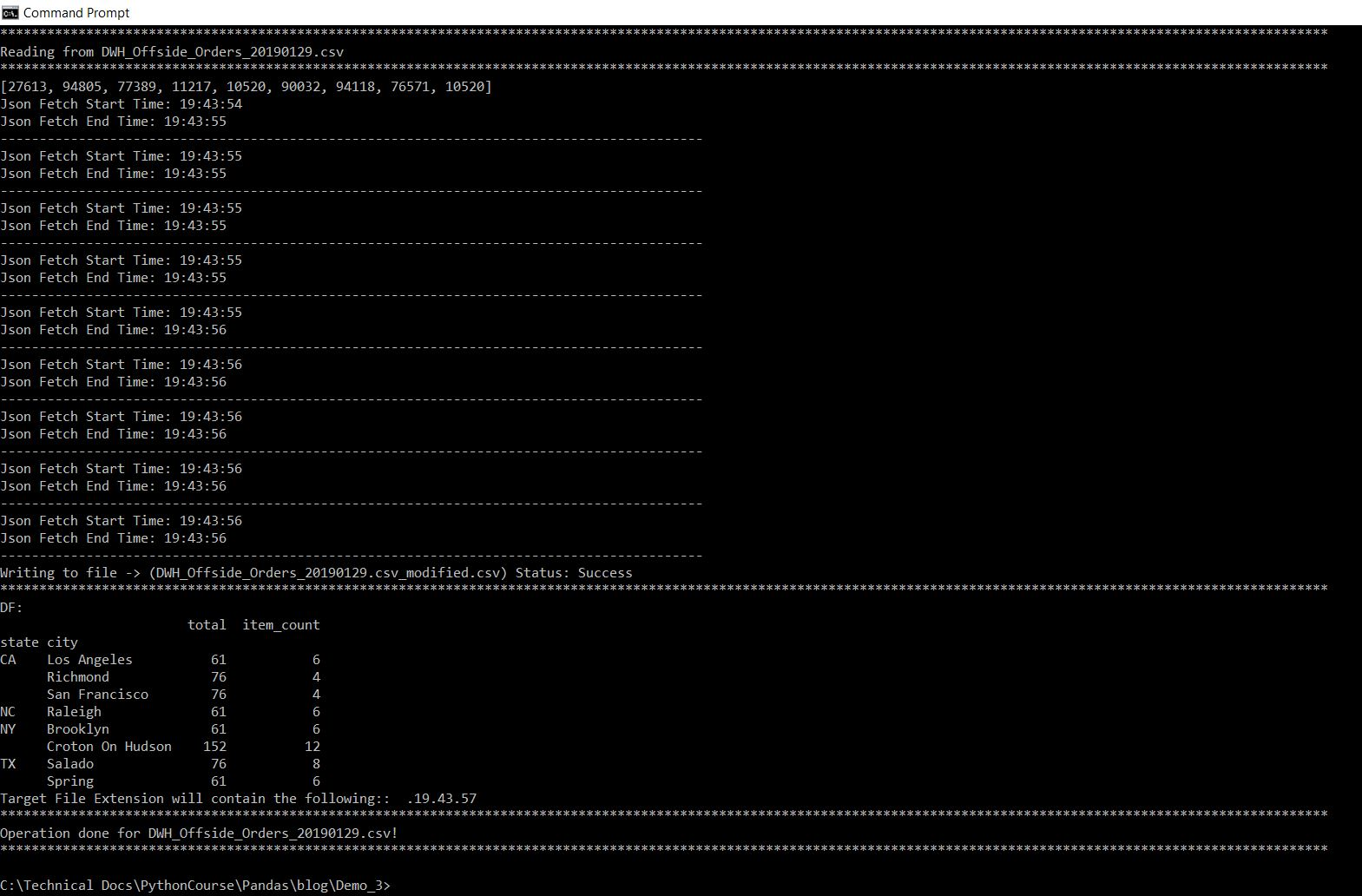Mac (32 bit):So, from the screenshot, we can see our desired output & you can calculate the aggregated value based on our sample provided in the previous screenshot.

Let’s check how the data directory looks like after run –

Windows: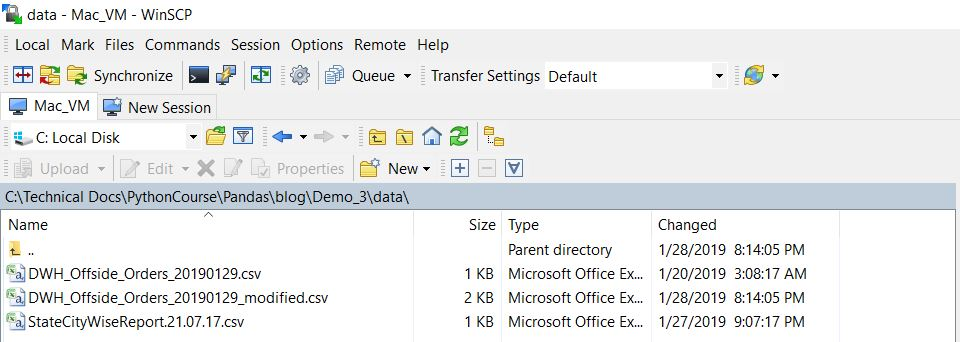MAC:So, finally, we’ve achieved our target.

I hope this will give you some more idea about more insights into the Python verse. Let me know – how do you think about this post.

Till then – Happy Avenging!